Home

ARTICLE 41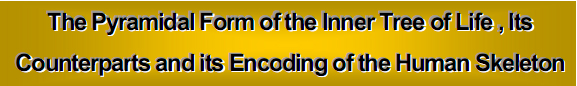by

Stephen M. Phillips

Flat 4, Oakwood House, 117-119 West Hill Road. Bournemouth. Dorset BH2 5PH. England.

Website: http://www.smphillips.mysite.com

Abstract

 The two-dimensional, inner form of the Tree of Life consists of two sets of seven regular polygons. Divided into their sectors, they become the projections of pyramids. 336 vertices, edges and triangles surround the vertical axes of each set. The seven pyramids therefore embody the structural parameter 336 of heterotic superstrings as the number of circularly polarized oscillations made by each closed curve of the superstring during one revolution around its axis. 16800 geometrical elements surround the axes of the two sets of 49 pyramids whose projections constitute the inner form of seven overlapping Trees of Life. They correspond to the 16800 circularly polarized oscillations in the ten closed curves of the superstring. The 80 vertices in the first four of the seven pyramids and the 126 vertices of the last three pyramids correspond to the 80 bones of the human axial skeleton and to the 126 bones of the appendicular skeleton. The 15 layers of polygons perpendicular to an axis joining two opposite A vertices of the disdyakis triacontahedron comprise 206 yods symbolizing the 206 bones of the human body. This is not coincidental, because the seven pyramids, the disdyakis triacontahedron, the tetrahedral, Platonic Lambda Tetractys and the inner form of ten overlapping Trees of Life are equivalent representations of holistic systems like the human body and the superstring. For example, the sum of the 20 integers in the tetrahedral Lambda is 350, and this is the number of geometrical elements surrounding the centres of the seven pyramids, the number of corners of the polygons enfolded in ten overlapping Trees of Life and the number of corners of the sectors of the 31 sets of polygons defined by the 31 axes joining opposite vertices of the disdyakis triacontahedron.

1. The 33 generic layers of vertices of the disdyakis triacontahedron

The disdyakis triacontahedron (Fig. 1) has seven parallel layers of vertices between diametrically opposite A vertices, 11 layers between opposite B vertices and 15  1  layers of vertices between opposite C vertices, totalling 33 types of layers, where 33 = 1! + 2! + 3! + 4!.2 The vertices in a layer are corners of a polygon whose centre lies on the line joining opposite vertices of the polyhedron. A sector of a polygon will be considered either as a single triangle (case a) or as divided into three triangles (case b).

Case a

Number of corners of triangles in the 33 layers = 6 + 10 + 15  +  62  = 93. (93–62=31) corners are not vertices of the polyhedron. This shows how the Godname EL with number value 31  prescribes the disdyakis triacontahedron. 90 corners surround the centre between  two opposite vertices. This property demonstrates that the disdyakis

1

triacontahedron is the polyhedral manifestation of Plato’s Lambda Tetractys (Fig. 1). The central number 6 denotes the six centres of the polygons formed by A vertices that surround the centre. There are 30 A vertices, 12 B vertices and 20 C vertices, so that

90 = 6 + 10 + 14 + (28+12+20, 30+10+20, or 30+12+18)

= 6 + 36  +  48,

where  48 = 28 + 20 or 30 + 18 and 36  = 10 + 14 + 12. The case where the axis passes through two opposite B axes is excluded for the sake of consistency with the Lambda Tetractys, i.e.,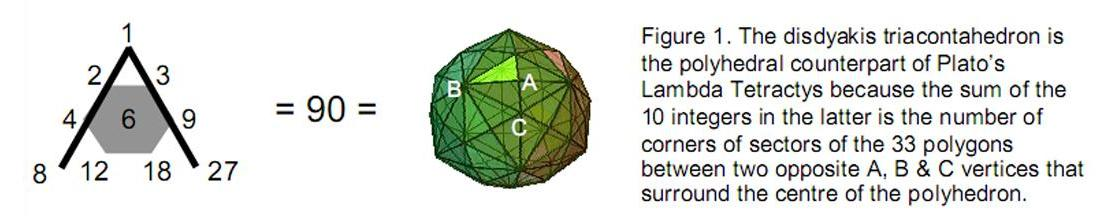the 90 corners must form sets of 48 and 36 — the respective sums of the six integers at the corners of the grey hexagon shown in Fig. 1 and the three integers at its corners. The following correspondences exist between the Lambda Tetractys and the disdyakis triacontahedron:

 Lambda Tetractys disdyakis triacontahedron 6 48 36 6 centres of polygons orthogonal to A-A axis 48 A & C vertices 36 corners either B vertices or centres of polygons orthogonal to B-B & C-C axes

92 vertices surround the centre of the polyhedron. The 46 vertices and their 46 mirror images have their counterpart in the 46 yods in each of the two triangles of the inner Tree of Life when their sectors are divided into three tetractyses (Fig. 2).Figure 2. The 46 yods in one triangle of the inner Tree of Life correspond to the 46 corners of the 33 polygons perpendicular to an A-A, B-B & C-C axis that surround the centre of the disdyakis triacontahedron. The 46 yods in the other triangle correspond to the mirror images of these 46 corners.

There are (7+2=9) vertices along an A-A axis, (11+2=13) vertices on a B-B axis and (15  +2=17) vertices on a C-C axis. The numbers of vertices surrounding the axes are:

 Axis: A-A B-B C-C 93 –9 93 –13 93 –17 84 80 76

84 vertices surround an A-A axis, where 84 = 12  + 32  + 52  + 72.  80 vertices surround a

2

B-B axis and 76  vertices surround a C-C axis, where 76  is the number value of YAHWEH ELOHIM, the Godname of Tiphareth.

Case b

60 sectors of polygons surround each A-A, B-B & C-C axis. At the centre of each sector is the corner of three triangles. The number of corners of triangles in the 33 layers = 93 + 60 + 60 + 60 = 273. There are (273–62 =211) corners other than vertices of the polyhedron. 210 (=21×10) corners surround the centre of the polyhedron. This shows how EHYEH, Godname of Kether with number value 21  , prescribes the disdyakis triacontahedron. 272  corners surround the centre, where

 272  = 2     4    6    8 10  12 14  16 18  20 22  24 26  28 30  32

is the number value of Cherubim  , the Order of Angels assigned to Yesod. 136 corners and their 136 mirror images surround the centre. This demonstrates how the Pythagorean Tetrad symbolized by the square determines the number of corners of the triangles making up the sectors of the 33 polygons making up the disdyakis triacontahedron. 136 corners belong to each half. Including the centre, there are 137 corners in each half of the polyhedron, each being mirror images of its counterpart in the other half. This is the number that approximates to the reciprocal of the fine-structure constant. The geometrical structure of the disdyakis triacontahedron therefore embodies one of the most important numbers in physics.

The Godname EL of Chesed with number value 31  prescribes the polyhedron because its 62  vertices comprise 31  pairs, one of which is diametrically opposite the other.

The number of corners of the triangles in the seven layers perpendicular to a straight line joining two diametrically opposite A vertices = 60 + 60 + 7 + 2 = 129. This is the number value of YAHWEH SABAOTH, the Godname of Netzach. YAHWEH, Godname of Chokmah, with number value 26  prescribes the 26  layers of vertices perpendicular to lines joining two diametrically opposite B or C vertices.

Now consider each of the 120 triangular faces of the disdyakis triacontahedron divided into three triangles. This adds one corner of a triangle per face, so that now there are (273+120=393) corners in case b (we need not consider case a because it would be inconsistent to treat sectors of polygons as single triangles but faces as divided into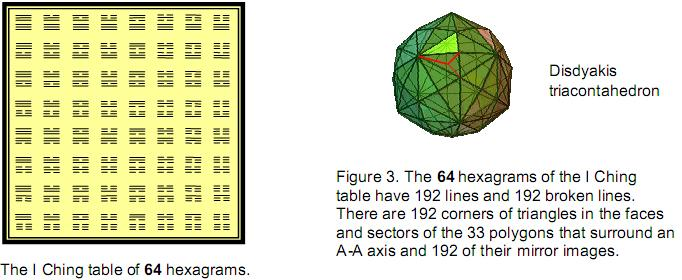3

three triangles). As nine vertices lie on an A-A axis, (393–9=384) corners surround this axis, i.e., 192 vertices and their mirror images. Compare this property with the 192 lines & broken lines in a half of the I Ching table and the 192 lines & broken lines in its other half (Fig. 3). The correspondence demonstrates that both are equivalent representations of a holistic system.

Of the 393 corners, 62  are vertices of the disdyakis triacontahedron. This is the number value of Tzadkiel  , the Archangel of Chesed.

In case b, the 15  polygons with 60 sides in the 15  ‘C’ layers have (62+60+15=137) corners, 136 corners surrounding the centre. The geometry of the disdyakis triacontahedron embodies the number 137 that is central to modern physics. Of these corners, (136– 62 =74) corners are not vertices of the polyhedron. The number of corners of the 11 polygons perpendicular to the line joining two opposite B vertices = 62  + 60 + 11 = 133. Of these, (133–62=71) are corners other than vertices, i.e., the triangles in the sectors of these 11 polygons in ‘B’ layers have 70 such corners surrounding the centre of the polyhedron. (74+70=144) corners of the (15+11=26) polygons other than vertices surround the centre. Including the 62  vertices, there are (144+62  =206) corners in these 26  layers that surround the centre. As the central ‘B’ and ‘C’ layers share the same centre, 25 corners in the 26  layers are centres of polygons (centre of the polyhedron+24 centres). There are (144–24=120) corners surrounding these 25 centres in the 26  layers, where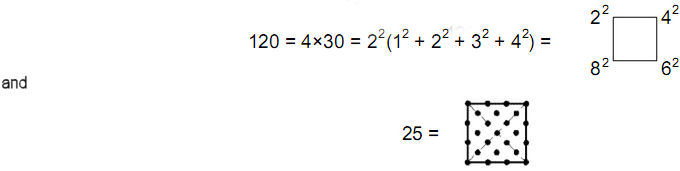It illustrates how the Tetrad (4), symbolized by the square, defines this property of two sets of layers of vertices of the disdyakis triacontahedron.

2. Properties of the seven polygons viewed as pyramid projections

Consider the inner form of the Tree of Life. As separate, regular polygons (Fig. 4), the equilateral triangle, square, pentagon hexagon, octagon, decagon & dodecagon have  48  corners.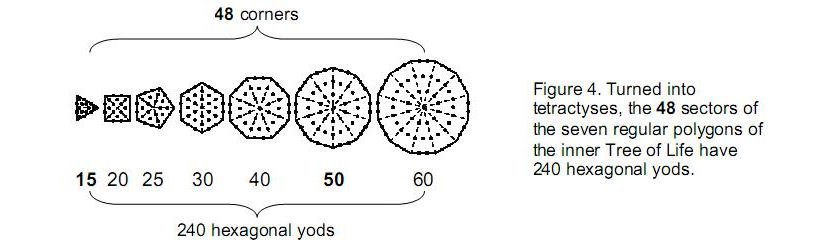Their 48 sectors have 55 corners, 96 edges & 55 triangles. These 48 sectors can be viewed as the 2-dimensional projection of pyramids. For example, the triangle divided into its three sectors is the projection of a tetrahedron and the square

4

with four sectors is the projection of a square-based pyramid. Each sector is the base of a tetrahedron. A pyramid with an n-sided regular polygon as its base (‘n-pyramid’) can therefore be divided equally into n tetrahedra that share the edge joining its apex to the centre of its base. Each inclined edge is the edge of an internal triangle. The internal and external triangles will be considered as either single triangles (case 1) or three triangles (case 2). The n-pyramid is made up of the following geometrical elements:

 Case 1 Case 2 Vertices: Sides: Triangles: n+2 3n+1 3n 4n+2 12n+1 9n Total = 7n+3 25n+3

Case 1

The seven pyramids have (48  + 7×2 = 62  vertices), (3×48  + 7 = 151) sides and (3×48  =144) triangles, totalling 357 geometrical elements. The number of elements surrounding the vertical axes of the pyramids (each comprising two vertices and one side) = 357 – 3×7 = 336, where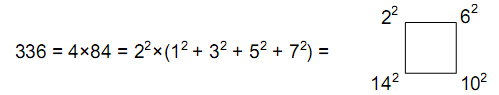This illustrates the Tetrad Principle. The elements consist of 48  vertices, 144 sides and 144 triangles. An n-pyramid has 7n elements surrounding its axis. As the first three polygons have 12 vertices, the next three polygons have 24 vertices and the last polygon has 12 vertices, the 336 geometrical elements comprise (7×12=84) elements for the first three pyramids, (7×24=168  ) elements for the next three pyramids and (7×12=84) elements for the last pyramid. In other words, 336 = 84 +168  + 84.

The cross pattée (Fig. 5) displays these numbers when its triangles are turned into 2nd-order tetractyses. Each 2nd-order tetractys contains 85 yods, i.e., 84 yods other than the apex that it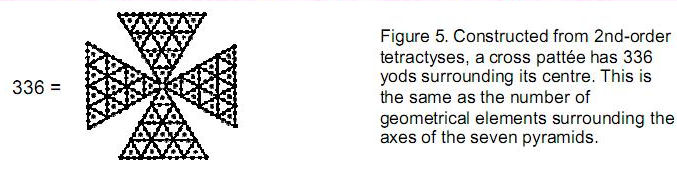shares with the three others. There are 84 such yods in the left-hand 2nd-order tetractys,
168  yods in the two, central 2nd-order tetractyses and 84 yods in the 2nd-order tetractys on the right-hand side.

The numbers of geometrical elements comprising the base of an n-pyramid and its upper part are:

 base upper   part Vertices: n+1 1 Sides: 2n n+1 Triangles: n 2n _____ _____ Total = 4n+1 3n+2

5

The 336 geometrical elements surrounding the axes of the seven pyramids consist of (4×48  =192) elements in their bases and (3×48  =144) above them. Starting from the 48  corners and 48  sides of the seven polygons, (3364848=240) extra geometrical elements are needed to transform them into pyramids. These elements correspond to the 240 hexagonal yods making up the seven separate polygons when their 48  sectors are turned into tetractyses (Fig. 4). They represent shape-determining degrees of freedom that are intrinsic to the seven Sephiroth of Construction symbolized by the seven hexagonal yods of each tetractys.

Case 2

The seven pyramids have 206 vertices, 192 of them surrounding their central axes.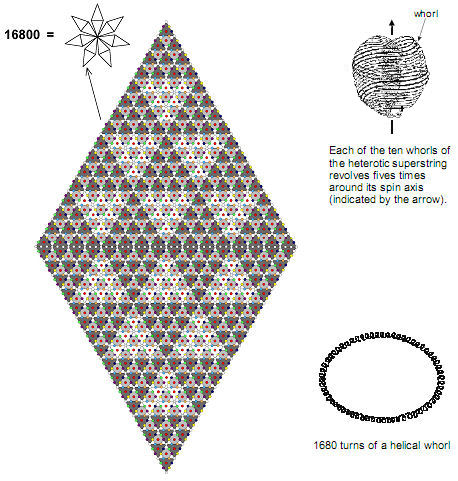Figure 6. Two 3rd-order tetractyses joined back-to-back form a parallelogram with 49  yods on each side.
The
49×49  array comprises 2401 yods. A star-shaped array of seven parallelograms has 16800 yods
surrounding its centre. They symbolize the 16800 turns of the ten helices making up the closed, heterotic
superstring constituent of up and down quarks.

6

They have 583 edges, of which 576 (= 242 = 12×22×32×42) surround their axes, and 432 triangles. There are 1221 geometrical elements, of which 1200 elements surround their seven axes. 2400 geometrical elements surround the 14 axes of the two identical sets of pyramids whose bases are the two sets of polygons. This number represents the reappearance of the Tree of Life parameter 240, which manifested in the earlier discussion of the seven separate polygons as the number of their hexagonal yods and as the extra number of geometrical elements needed to transform them into pyramids.

The numbers of geometrical comprising the base of an n-pyramid and its upper part are:

 base upper part total Vertices: 2n+1 2n+1 4n+2 Sides: 5n 7n+1 12n+1 Triangles: 3n 6n 9n ______ ______ ______ Total = 10n+1 15n+2 25n+3

(10×48=480) elements in the bases of the seven pyramids surround their centres and (15×  48=720) elements in their upper parts surround their axes. The 480 elements comprise 240 sides and 240 vertices and triangles. This is the counterpart of the 240 hexagonal yods in each of the two identical sets of seven regular polygons. It is also the counterpart of the (240+240) roots of the heterotic superstring symmetry group E8×E8.

Enfolded in each tree of a set of overlapping Trees of Life are the two sets of seven polygons. Each is the projection of a pyramid. As found earlier, 1200 geometrical elements surround the axes of a set of seven pyramids associated with each tree. Each of the two sets of (7×7=49) separate pyramids associated with seven overlapping Trees of Life has (7×1200=8400) geometrical elements surrounding their axes. The two sets therefore have (2×8400=16800) such elements. This 8400:8400 division of elements is the counterpart of the 8400 circular turns of the ten helical whorls in the inner and outer halves of the UPA/heterotic superstring. Each tree is a representation of a Sephirah of Construction and so the 16800 helical turns of the superstring are the 3-dimensional counterpart of these geometrical elements as bits of information needed for the Sephirothic manifestation of the cosmic blueprint called the Tree of Life.

A 3rd-order tetractys has 49 yods on each side. Two 3rd-order tetractyses laid back-toback forms a 49×49 parallelogram array of (492=2401) yods. Seven such parallelograms joined at one corner to form a star have (7×2400=16800) yods surrounding its centre (Fig. 6). This is the remarkable counterpart of seven overlapping Trees of Life, enfolded in each of which are 14 regular polygons — the projections of 14 pyramids that have 2400 geometrical elements surrounding their axes.

EL CHAI, the Godname of Yesod, has the number value 49. It prescribes these Tree of Life and tetractys representations of the superstring structural parameter 16800. EL, the Godname of Chesed, prescribes the parallelogram because 31 tetractyses lie along each side. Each half-parallelogram comprises

1 + 3 + 5 + 7 + … + 31   = 162  = 44  = 256

tetractyses. The 16800 yods belong to (2×256=512) tetractyses, where

 512 = 2  6 10  14 18 22 26  30 34 38 42 46 50  54 58 62

7

Once again, this illustrates how the Pythagorean Tetrad mathematically defines the properties of archetypal representations of numbers of universal significance (here, the 16800 circularly polarised oscillations in the standing waves running around the ten closed curves of the heterotic superstring).

Now let us turn into tetractyses all the triangles making up the seven pyramids in case 1. An n-pyramid has (n+2) yods at vertices, 2 hexagonal yods along each of the L edges and one yod at the centre of each of its T triangles. As L = (3n+1) and T = 3n, the number of yods in an n-pyramid = n + 2 + 2(3n+1) + 3n = 10n + 4. The number ‘4’ denotes the four yods on the central axis. There are (10×48  =480) yods surrounding the axes of the seven pyramids (240 yods in the first three pyramids & last pyramid, 240 yods in the 4th, 5th & 6th pyramids). As found earlier, this is the same as the number of geometrical elements in the bases of the pyramids surrounding their centres. There are also 480 hexagonal yods in the two sets of seven separate polygons, 240 per set (see Fig. 4). The (240+240) yods surrounding the axes of the seven pyramids symbolize the (240+240) roots of E8×E8  , the symmetry group of the E8×E8  heterotic superstring.

For case 1, the number of yods on the edges of the 3n triangles in an n-pyramid = n + 2 + 2L = 7n + 4. The number of yods on the (3×48  =144) edges of the 144 triangles of the pyramids surrounding their axes = 7×48 =336. This is the number of geometrical elements surrounding the axes of the pyramids in case 1. Once again, the form-defining character of the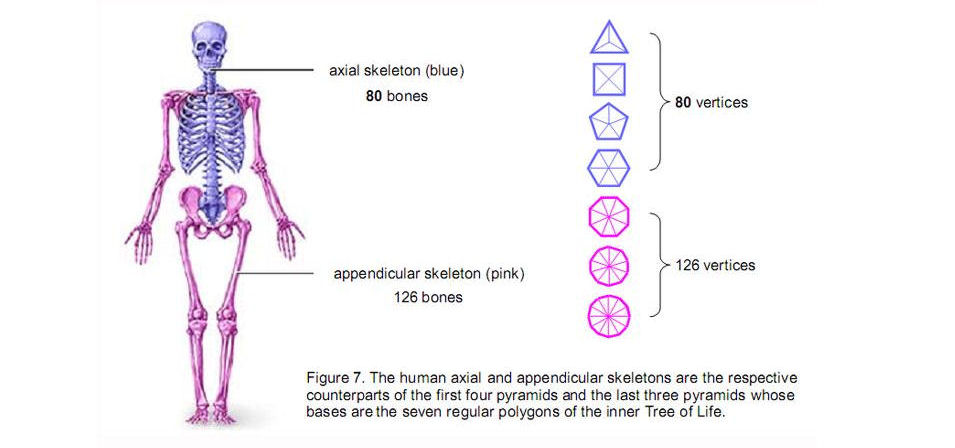number 336 in shaping the seven pyramids is revealed. In the context of their microscopic realisation in the E8×E8 heterotic superstring, this is the number of helical turns made by every whorl of this superstring during each of the five revolutions around its axis of spin (see Fig. 6).

3. The human skeletal counterpart of the seven pyramids

For case 2, an n-pyramid has (4n+2) vertices. The seven pyramids have (4×48  + 7×2 = 206) vertices, the first four pyramids with 18 corners of its bases have (4×18 + 4×2 = 80)  vertices and the remaining three pyramids have 126 vertices. This 80  :126 division of vertices between the first four and the last three pyramids has its counterpart in the

8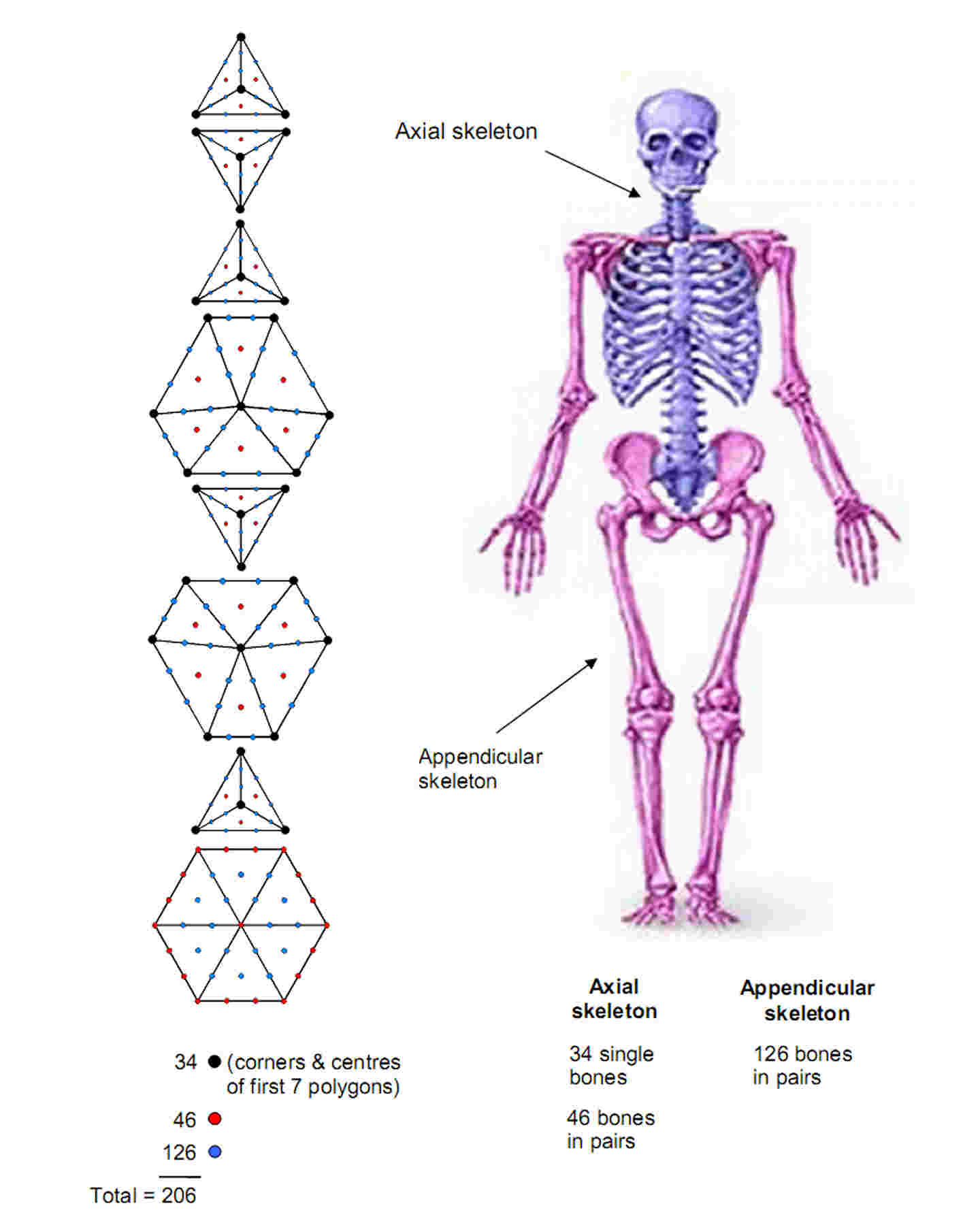Figure 8. Constructed from tetractyses, the eight types of polygons perpendicular to a C-C axis that are formed by vertices of the disdyakis triacontahedron have 206 yods. They symbolize the 206 bones in the human skeleton. The 34 yods at corners and centres of the first seven polygons symbolize the 34 single bones of the axial skeleton. The remaining 172 yods denote the 172 bones that exist as pairs.

9

human body as the 80  bones of the axial skeleton and the 126 bones of the appendicular skeleton (Fig. 7). The first four pyramids correspond to the axial skeleton and the three remaining pyramids correspond to the appendicular skeleton. It is readily verified that no other subset of pyramids has 80  or 126 vertices.

The axial skeleton is the basic core of the human skeleton, protecting the vital organs. The 26  vertices at the corners and centres of the bases of the four pyramids or at their apices symbolize the 26   vertebrae. They are prescribed by the Godname YAHWEH. The eight vertices at the centres of the faces of the triangle and pentagon symbolize the remaining eight single bones of the appendicular skeleton. The remaining 46 vertices of the first four pyramids denote the 23 pairs of bones of the axial skeleton. The 126 vertices of the last three pyramids denote the 126 bones of the appendicular skeleton.

4. The disdyakis triacontahedron encodes the human skeleton

Perpendicular to the axis joining diametrically opposite C vertices of the disdyakis triacontahedron are 15  layers of polygons. They are of eight types (Fig. 8). Constructed from tetractyses, they comprise 206 yods. The 34 corners and centres of the first seven types of polygons above the hexagon in the equatorial plane symbolize the 34 bones of the axial skeleton and the 172 other yods denote the 172 bones of the axial and appendicular skeletons that exist in the human body as pairs.

We found earlier for case 1 that the 336 geometrical elements surrounding the axes of the seven pyramids comprise (7×12=84) elements for the first three pyramids, (7×24=168) elements for the next three pyramids and (7×12=84) elements for the last pyramid. We also saw that this 84:168  :84 pattern manifests in cross pattée when its four triangular arms are turned into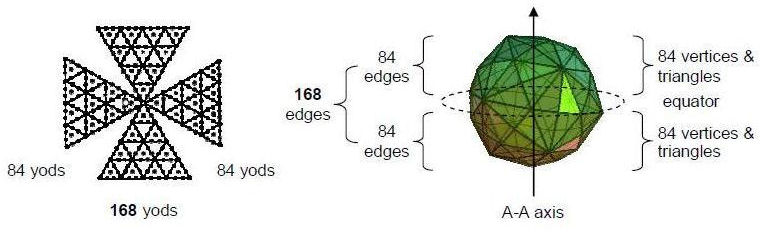Figure 9. The cross pattée displays the same 84: 168:84 pattern in the distribution of yods about its centre as the disdyakis triacontahedron does
in the distribution of geometrical elements about its equator.

2nd-order tetractyses. We will now show that the same pattern appears in the disdyakis triacontahedron when its vertical axis is a straight line joining two diametrically opposite A vertices. This polyhedron has 60 vertices, 180 edges and 120 triangular faces (360 geometrical elements) surrounding any axis passing through two diametrically opposite vertices. There are seven sheets of vertices perpendicular to an A-A axis between two opposite A vertices. The fourth polygon forming the equatorial plane has 12 vertices and 12 edges, totalling 24 geometrical elements. (360–24=336) elements lie above and below the equator, 168  on each side. As the polyhedron has 180 edges, there are (180–12=168)  edges above and below its equator, 84 on either side. There are 48  vertices above and below the equator, 24 vertices on either side. 60 triangles are on either side of the equator. The 168

10

geometrical elements on each side comprise 84 edges and (24+60=84) vertices and triangles. The disdyakis triacontahedron therefore displays 168 edges and one set of 84 vertices and triangles above and below its equator. This is the same 84:168:84 pattern as that displayed by the seven pyramids and by the cross pattée (Fig. 9). The centre of the cross, the axes of the seven pyramids and the 12 edges and 12 vertices in the equator of the disdyakis triacontahedron are counterparts in this correspondence.

When the 120 triangular faces of the disdyakis triacontahedron are turned into tetractyses, (2×180=360) hexagonal yods must be added to its 180 edges. In the orientation where an A-A axis is vertical, 12 vertices and 12 edges lie in the equatorial plane. This leaves 84 edges above the plane and 84 edges below it. (2×84=168) yods have to be added to each set. 336 hexagonal yods have to be added to create the edges of the polyhedron above and below its equator. There are 168 hexagonal yods on the edges above the equator and 168 of their inverted counterparts below it. The 12 edges in the equator comprise four A-B edges, four A-C edges & four B-C edges. The polyhedron has 60 of each type. Therefore, there are 28 of each type above the equator and 28 of each type below it. Each edge has two hexagonal yods, so the 168 hexagonal yods in either half of the polyhedron consist of two sets of three, a set comprising a yod on 28 (A-B), 28 (A-C) & 28 (B-C) edges. The pairing of hexagonal yods on each edge creates the 84:84 division, whilst the inversion symmetry of the polyhedron creates the 168:168 division.

The construction of the disdyakis triacontahedron from tetractyses requires 360 yods on its edges, 180 for each half, and 120 yods at the centres of its faces, 60 for each half. (180+60=240) new yods are required for each half. This is the polyhedral counterpart of the 240 yods surrounding the axes of the first three pyramids and the last one and the 240 yods surrounding the axes of the fourth, fifth & sixth pyramids. It is also the counterpart of the 240 extra geometrical elements needed to turn each set of seven regular polygons into pyramids and the counterpart of the 240 hexagonal yods in each set of polygons. The 240:240 division appears in all forms of sacred geometry because they embody the gauge group symmetry E8×E8 of superstring forces, wherein E8 has 240 non-zero roots.

5. The disdyakis triacontahedron as the tetrahedral Lambda Tetractys

The disdyakis triacontahedron has 30 A vertices, 12 B vertices and 20 C vertices. Perpendicular to each of the 15  A-A axes are seven sheets of polygons with 60 vertices and (60+7=67) corners of their 60 sectors. Perpendicular to each of the 6 B-B axes are 11 sheets of polygons with 60 vertices and (60+11=71) corners of their 60 sectors. Perpendicular to each of the 10 C-C axes are 15  sheets of polygons with 60 vertices and (60+15=75) corners of their 60 sectors. There are (15×7 + 6×11 + 10×15  = 321) polygons and (15+6+10=31) axes. The Godname EL with number value 31  prescribes the number of axes defining sets of polygons created by the vertices of the disdyakis triacontahedron. As there are three polygons on each side of an A-A axis and as the centre of the middle polygon coincides with the centre of the middle polygon for every other axis, the 15 A-A axes define (15×7=105) polygons with (15×6 + 1 = 91) different centres, i.e., 90 centres are distributed about the centre of the polyhedron. The (6×11=66) polygons perpendicular to B-B axes have (6×10=60) centres distributed around the centre of the polyhedron. The (10×15=150) polygons perpendicular to C-C axes have (10×14=140) centres arranged around the centre of the polyhedron. Hence, there are (91+60+140=291) centres of polygons. Including the 62  vertices of the polyhedron, the triangular sectors of the 321 polygons have (291+62=353) corners. 350 corners other than two opposite vertices surround the centre of the disdyakis

11

triacontahedron. They comprise the 90 centres of the 105 polygons perpendicular to the 15 A-A axes, the (60+140=200) centres of the (66+150=216) polygons perpendicular to either B-B or C-C axes and the 60 polyhedral vertices surrounding any axis.

The 350 corners split up into 90 centres of polygons perpendicular to the 15 A-A axes and (200+60=260) corners of sectors of polygons perpendicular to the (6+10=16) B-B and C-C axes. This 15:16 division is found in the pentagon constructed from tetractyses (Fig. 10). 15 yods lie on its boundary, which encloses 16 yods. The Godname YAH with number value 15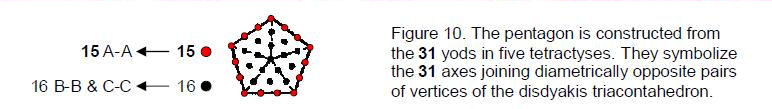prescribes this division of the 31 yods, which are prescribed by the Godname EL with number value 31. The yods along the boundary signify the A-A axes and the internal yods symbolize the B-B and C-C axes.

The 90:260 division of the number 350 manifests in the tetrahedral version of Plato’s Lambda tetractys3 (Fig. 11). The sum of the 10 numbers arranged in a tetractys and forming one face of the tetrahedron is 90. The sum of the other 10 numbers is 260. This is no coincidence, because both the tetrahedral Lambda and the disdyakis triacontahedron are archetypal representations of holistic systems, one arithmetic and the other geometrical.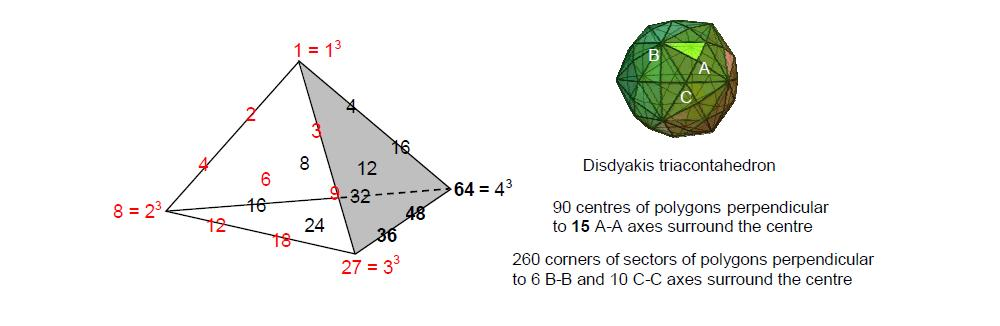Figure 11. The sum of the 10 red numbers of Plato’s Lambda Tetractys is 90. The sum of the 10 remaining numbers in the tetrahedron is 260. The disdyakis triacontahedron is the polyhedral counterpart of this archetypal arrangement of integers because the 105 polygons perpendicular to the 15 A-A axes have 90 different centres surrounding its centre and the 216 polygons perpendicular to the 6 B-B & 10 C-C axes have 260 centres and corners surrounding its centre.

This is further indicated by the fact that the number 6 at the centre of the Lambda tetractys denotes the six centres of the polygons perpendicular to an A-A axis above and below the centre of the polyhedron, whilst the sum 84 of the nine other integers in this tetractys is the number of similar centres of the 84 polygons perpendicular to the 14 other axes ( 15  polygons share the same centre). Musically speaking, the number 6 at the centre of the tetractys represents the tonic of the Pythagorean scale because, when all the integers are divided by 6, all the resulting ratios surrounding 1 are the tone ratios of notes of different octaves of this scale. There are 28 intervals between the eight notes of one octave and eight unit intervals, making 36  intervals. Ten octaves comprise 71 notes for which there are 71 unit intervals and

12

ten repetitions of the 28 intervals between the eight notes in each octave, i.e., (71 + 10×28 = 351) intervals. There are 350 intervals up to the tenth octave, excluding the unit interval between the tenth octave and itself. They are symbolized by the 350 corners of the sectors of the 321 polygons that surround the centre of the disdyakis triacontahedron.

The seven enfolded polygons in the inner Tree of Life have 36 corners. For a set of overlapping Trees of Life, each tree has a similar inner form. As the topmost corner of the hexagon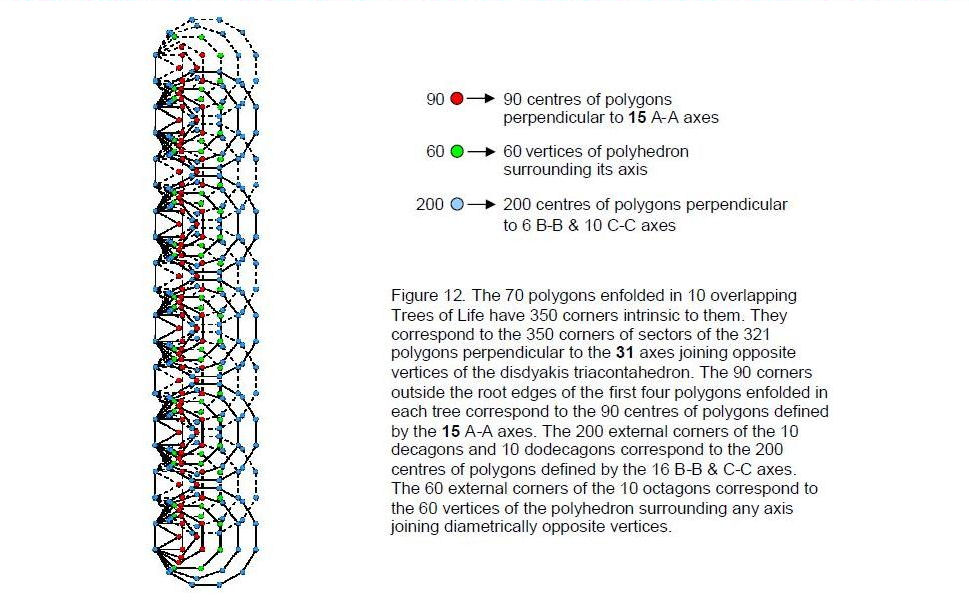associated with any tree coincides with the lowest corner of the hexagon in the next higher tree, the number of corners of the 7n polygons enfolded in n overlapping Trees of Life is 35n + 1. The 351 corners of the 70 polygons enfolded in 10 such trees comprise the 91 corners of the triangle, square, pentagon and hexagon in each tree outside their root edges, the 60 external corners of the octagons and the 200 corners of the decagons and dodecagons (Fig. 12). As the topmost corner of the hexagon enfolded in the tenth tree coincides with the lowest corner of the hexagon enfolded in the eleventh tree, there are intrinsic to ten trees 350 corners comprising 90 corners of the first four polygons and 60 corners of the octagons and 200 corners of the decagons and dodecagons. Compare this with the disdyakis triacontahedron, whose centre is surrounded by 350 corners of the sectors of polygons comprising the 90 corners defined by A-A axes, the 60 polyhedral corners and the 200 corners defined by B-B and C-C axes. The correlation demonstrates that the disdyakis triacontahedron is the polyhedral counterpart of the inner form of the ten overlapping Trees of Life, each tree signifying a Sephirah. This further demonstrates its holistic character.

It was found in Section 2 that the seven pyramids are composed of 357 geometrical

13

elements. 350 elements surround the centres of their bases. The number of bits of geometrical information needed, starting with their centres, to express their shapes is exactly equal to the number of corners marking the shapes of the 70 regular polygons enfolded in 10 overlapping Trees of Life, to the number of corners of sectors of all the polygons formed by the vertices of the disdyakis triacontahedron and to the sum of the 20 integers of the tetrahedral Lambda. This correspondence exists because they are all manifestations of the same mathematical archetype governing representations of holistic systems. Surrounding the centre of the base of the n-pyramid are (7n+2) geometrical elements. The 3-, 4- & 5-pyramids have [7×(3+4+5) + 3×2 = 90] elements, so that the 6-, 8-, 10- & 12-pyramids have 260 elements.

The following remarkable correspondences exist between these four different representations of holistic systems:

 Tetrahedral Lambda Tetractys 7 pyramids inner form of 10 Trees of Life disdyakis triacontahedron Sum of 10 integers in first face = 90. Sum of 10 integers in other 3 faces = 260. Sum = 350 90 geometrical elements surround centres of first 3.260 elements surround centres of last 4. Total = 350 elements 90 corners of first 4 polygons outside root edges. 260 corners of last 3 polygons. Total = 350 corners 90 centres of polygons perpendicular to A-A axes. 260 corners of sectors of polygons perpendicular to B-B & C-C axes. Total = 350 corners

The 90:260 division occurring in these representations has the following arithmetic counterpart. As

90 = 2 + 3 + 4 + …+ 13,

90 is the sum of the 12 integers after 1. As

350 = 2 + 3 + 4 +… + 26  ,

350 is the sum of the 25 integers after 1. Symbolizing the Tetrad (4), the square represents these two numbers when it is constructed from four tetractys arrays of the 25 integers 2–26 (Fig. 13). The sum of the 12 integers on the edges of the square is 90 and the sum of the 13 integers inside it is 260. The largest (central) integer in the square array is 26, the number value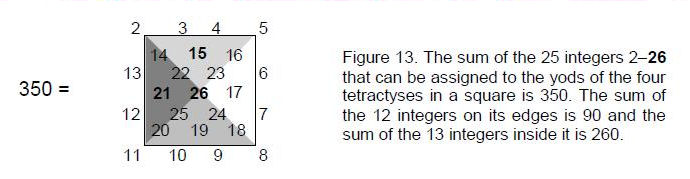of YAHWEH, the Godname of Chokmah. This demonstrates the archetypal character of the number 350 as the number of degrees of freedom that are needed to generate the complete form of a representation of a holistic system. Instead of a square divided into its sectors, Fig. 13 can depict a pyramid. It is the square pyramid — no other one — that can represent this archetypal number when the integers after 1 are assigned to the positions of the yods making up the tetractyses that form its faces. It may be thought of as an alternative representation in terms of

14

integers to the tetrahedral Lambda Tetractys in which the boundary of the square corresponds to the first face of the tetrahedral array of 20 integers and its interior corresponds to the three other faces. The inner Tree of Life consists of two identical sets of seven regular polygons, enfolded in one another so that they share the same root edge. The polygons in one set are the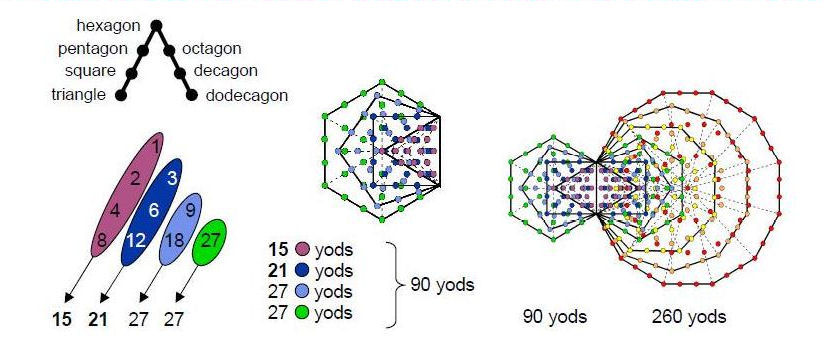Figure 14. The sums of the four diagonal rows of integers in the Lambda Tetractys are the numbers of yods outside the root edge in the first four polygons of the inner Tree of Life. The sum of the remaining 10 integers of the tetrahedral Lambda is 260. This is the number of yods outside the root edge in the seven polygons.

mirror images of their counterparts in the other set. The first four enfolded polygons have 90 yods outside their shared, root edge (Fig. 14). The seven enfolded polygons have 260 yods outside their shared edge. This demonstrates the power of the Tetrad to define sections of the inner Tree of Life whose properties correspond to other representations of holistic systems.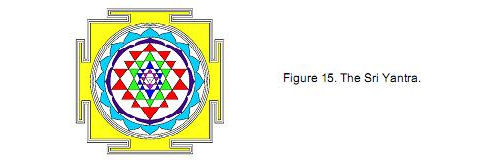It is not plausible that this is coincidence because the 15, 21, 27 & 27 yods in, respectively, the triangle, square, pentagon and hexagon outside their shared root edge correspond to the sums of the diagonal rows of integers in the Lambda Tetractys! The square with 21 external yods and the dodecagon with 69 external yods also have 90 yods outside the root edge. However, the numbers 15 and 27 do not appear in a natural way in terms of the yods in the tetractys-divided dodecagon. Here, therefore, is the unique, single, inner Tree of Life counterpart to the four previously discussed divisions of the number 350 into 90 and 260.

15

6. The Sri Yantra as the tetrahedral Lambda Tetractys

The Tantric Sri Yantra4 consists of 42 triangles (Fig. 15) in four sheets distributed around a central triangle with a dot (bindu) at its centre. It is generated from nine triangles — five pointing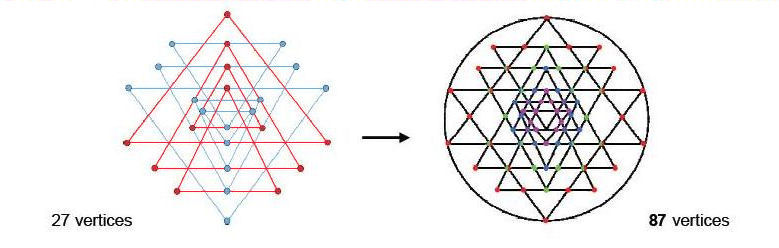Figure 16. Five downward-pointing blue triangles and four upward pointing red triangles create the 43 triangles of the Sri Yantra. ( Coloured half circles denote vertices belonging to adjacent triangles )

downwards representing the feminine (Shakti) principle and four pointing upwards representing the masculine (Shiva) principle (Fig. 16). The overlapping of these nine triangles creates 43 triangles with 87 vertices surrounding the central bindu. 87 is the number value of Levanah, the Mundane Chakra of Yesod. 84 vertices surround the central triangle, where

84 = 12  + 32 + 52  + 72.

According to Table 1, there are 260 geometrical elements in the Sri Yantra, showing how the Godname YAHWEH with number value 26 prescribes the Tantric representation of divine creation. The 43 triangles have 129 edges, showing how the shape of the Sri Yantra is prescribed by the Godname.

Table 1. Geometrical composition of the Sri Yantra.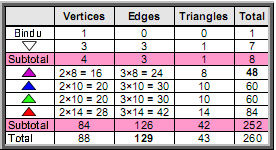Now consider each of the nine primary triangles as divided into three triangles. The (9×3=27) new triangles have (9×4=36) vertices and (9×6=54) edges, that is, 117 geometrical elements. Their outer 27 vertices are shared with the 43 triangles of the Sri Yantra, leaving 9 unshared vertices (the centres of the triangles). Hence, (9+54+27=90) geometrical elements are intrinsic to the nine generating triangles in the sense they are not also elements making up the 43 triangles of the Sri Yantra. Their nine centres consist of eight centres of four pairs of mutually inverted triangles and one centre of a Shakti triangle that is unpaired with a Shiva triangle. Compare this with the 10 integers

16

of the Lambda Tetractys (Fig. 17). The integer 1 corresponds to the centre of the unpaired, Shakti triangle and the integer 8 corresponds to the eight centres of the four pairs of Shiva and Shakti triangles. The number 27 at the third corner of the tetractys corresponds to the 27 triangles making up the nine primary triangles. The sum of the seven integers located at the centre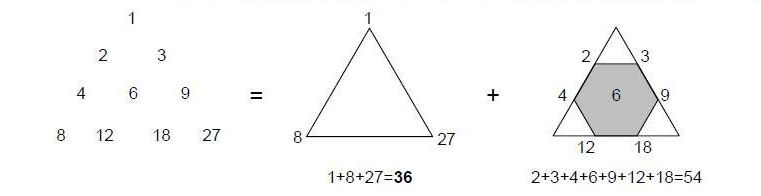Figure 17. The sum of the integers at the corners of the Lambda Tetractys is 36  . This is the number of triangles (27) and unshared vertices (9) of the 27 triangles making up the nine primary triangles that generate the Sri Yantra. The sum of the remaining integers at the centre and corners of the grey hexagon is 54. This is the number of edges of the 27 triangles. The nine primary triangles conform to the 36  :54 division of the Lambda Tetractys.

and corners of a hexagon is 54. This corresponds to the 54 edges of the 27 triangles. We find that the pattern of geometrical elements making up the nine triangles that are not shared with the 43 triangles of the Sri Yantra conforms to the archetypal array of integers in the Lambda Tetractys. This is hardly surprising, as the nine triangles, as the progenitor of a holistic system of sacred geometry, must themselves exhibit holistic characteristics.

The geometrical composition of the nine primary triangles and the 43 triangles of the Sri Yantra is shown below:

 vertices edges triangles Total Intrinsic to nine triangles: 9 54 27 90 Sri Yantra: 88 129 43 260 Total = 97 183 70 350

They are made of 350 geometrical elements, of which 90 belong to the nine triangles and 260 belong to the 43 triangles of the Sri Yantra. This 90:260 division is identical to

Table 2. The number values of the 10 Sephiroth in the four Worlds.

 Sephirah Title Godname Archangel Order of Angels Mundane Chakra Kether 620 21 314 833 636 Chokmah 73 15, 26 248 187 140 Binah 67 50 311 282 317 Chesed 72 31 62 428 194 Geburah 216 36 131 630 95 Tiphareth 1081 76 101 140 640 Netzach 148 129 97 1260 64 Hod 15 153 311 112 48 Yesod 80 49 246 272 87 Malkuth 496 65, 155 280 351 168

17

that found in Section 5 for the sum of the 20 integers of the tetrahedral Lambda Tetractys. Just as the 10 integers adding to 90 in the first face of this array generate the 10 integers adding to 260 of the three remaining faces, so there are 90 geometrical elements intrinsic to the nine primary triangles, which generate the Sri Yantra with 260 geometrical elements. They have (97+183=280) vertices and edges, where 280 is the number value of Sandalphon, Archangel of Malkuth. EL CHAI, Godname of Yesod with number value 49, prescribes the nine primary triangles and the Sri Yantra because they have 97 vertices, where 97 is the 49th odd integer. 97 is also the sum of the Godnames of Kether, Chokmah & Binah, the Sephiroth belonging to the Supernal Triad:

21  +  26  +  50  = 97.

97 is also the number value of Haniel, the Archangel of Netzach. Similarly, we found that there are 90 centres of the 105 polygons perpendicular to the 15 A-A axes of the disdyakis triacontahedron that surround its centre, whilst 260 centres and vertices of the 216 polygons perpendicular to the six B-B and 10 C-C axes surround its centre. Then we saw in Section 2 that the seven pyramids, whose bases are the seven regular polygons of the inner Tree of Life, have 350 geometrical elements surrounding the centres of their bases, the first three pyramids having 90 elements and the last four pyramids having 260 elements. Finally, we found in Section 5 that the first four polygons enfolded in 10 overlapping Trees of Life have 90 corners outside their shared edges apart from the highest corner of the hexagon in the tenth tree, which coincides with the lowest corner of the hexagon in the eleventh tree. The last three polygons enfolded in each of the 10 trees have 260 corners.

7. Conclusion

The tetrahedral Lambda Tetractys, the disdyakis triacontahedron, the inner form of 10 Trees of Life, the seven pyramids and the nine triangles that generate the Sri Yantra are all isomorphic representations of a holistic system embodying the divine archetypes that are implemented by the Godnames.

References

1. Numbers highlighted in boldface are the number values of the Sephiroth, their Godnames, Archangels, Orders of Angels and Mundane Chakras. They are listed in Table 2 above.

2. Phillips, Stephen M. Article 25: “The 33 Vertex Sheets of the Disdyakis Triacontahedron Signify the 33 Tree Levels of Ten Overlapping Trees of Life,” (WEB, PDF), p. 12.

3. Phillips, Stephen M. Article 11: “Plato’s Lambda — Its Meaning, Generalisation and Connection to the Tree of Life,” (WEB, PDF), pp. 7–9.

4. Phillips, Stephen M. Article 35: “The Tree of Life Nature of the Sri Yantra and Some of its Scientific Meanings,” (WEB, PDF), p. 1 et seq.

18

Home